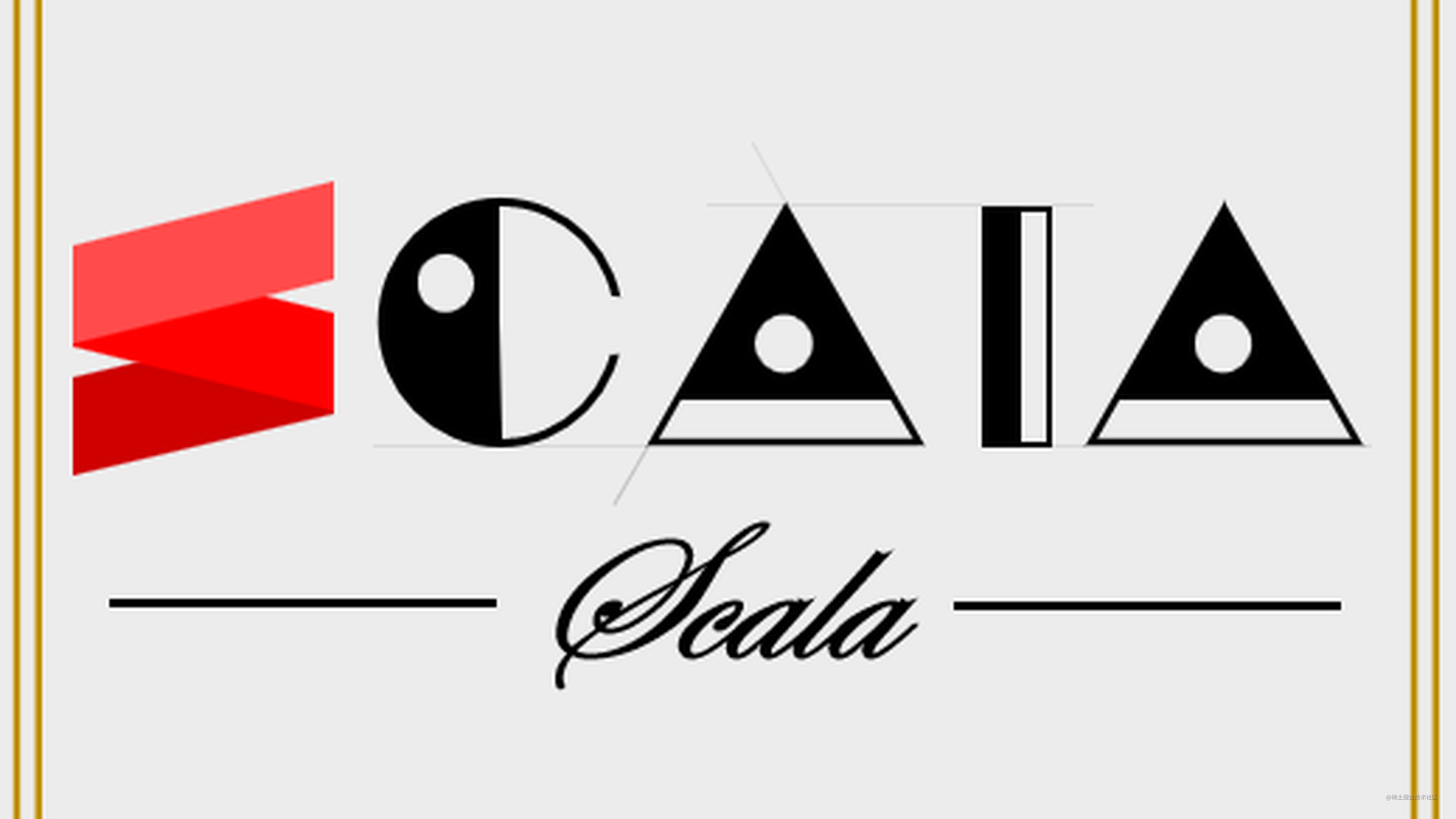# Scala ：Function as ValueOOP，Object Oriented Programming，面向对象编程，它的核心思想是：

• 封装状态 state，让系统中的各个组件保持有限的通信与访问，同时各司其职，降低耦合。OOP 适用于通过大量内部变量来维护状态的复杂系统。

FP，Funtional Programming，面向函数编程 ( 或称函数式编程 )，它的核心思想是：

• 封装行为 action ，推崇简单的行为去组合，描述复杂的行为。FP 适用于无状态的，基于数据流管道执行纯计算任务的系统。

Scala 是一门多范式的语言，OOP 和 FP 在这里可以共存，乃至相辅相成。因此，面对不同的设计任务时，Scala 开发者仍能自由地切换最合适的编程范式去解决问题。Scala 的函数有更丰富的语义，了解它们是之后学习并投入到函数式编程的基础。

``````val f : Int => Int = (a : Int) => 2 * a     // f(a) = 2a

``````val f1 : Int => Int = a => 2 * a     // f(a) = 2a
val f2 : Int => Int = 2 * _
val f3 = 2 * (_ : Int)

Scala 使用 函数标识符 来表示传递函数本身，而非调用。有时为了避免歧义，也可以在标识符后面加上 `_`。如：

``````def f(a : Int) = 2 * a
// val g : Int => Int = f _
val g: Int => Int = f
g(10) // == f(10)

## 传名调用与传值调用

Scala 默认：当调用某个函数时，入参要被率先求值，这个行为称 传值调用 call by value。比如：

``````def foo(a : Int) = 2 * a
def goo(b : Int) = 3 * b
// 首先调用 goo，然后调用 foo
foo(goo(1))

``````def foo(a : => Int) = 2 * a
def goo(b : Int) = 3 * b
// 首先调用 foo， 然后调用 goo
foo(goo(3))

## 副作用与纯函数

``````var global = 0
def f() : Unit = global = global + 1

``````def g(a : Int, b : Int) = a + b

## 统一访问原则

Scala 允许声明不带任何参数列表的 无参数函数。形如：

``````def str : String = "hello world"

``````val str : String = "hello world"

``````val bill = new Trolley(amount = 10,price = 2,coupon = 0.8)
bill.total

``````class Trolley(amount : Int,price : Int, coupon : Double) {
def total: Double = amount * price * coupon
}

``````def g1() : Int = 100
def g2   : Int = 100

``````println(s"value of invoke g1() : \${g1()}")
println(s"value of invoke g2   : \${g2}")

## 闭包与高阶函数

``````var y = 0
def g(x : Int): Int = x + y

``````val closure: Unit = {
var y = 0
def g(x : Int): Int = x + y
}

``````def hof(f: (Int, Int) => Int, a: Int, b: Int): Int = f(a,b)

``````def context(x : Int, y : Int) : Int = {
def run(a : Int) : Int = x * y - a
run(10)
}

`x``y` 对于 `run` 而言是自由变量。如果只从 `run` 本身分析，它是一个非纯函数，因为它的返回值不完全取决于它的参数列表。但从高阶函数 `context` 的作用域分析，`context` 包含了计算 `run` 所需的信息。比如给定 `context(x = 1, y = 2)``run` 的所有自由变量转为常量，此时它可被认为是纯函数。由此还可知，函数的纯粹性是在一个上下文中去讨论的。

``````def action (f : () => Unit): Unit = f()
action {()=>
val a = 1
val b = 1
println(a + b)
}

``````def action (f : => Unit): Unit = f
action {
val a = 1
val b = 1
println(a + b)
}

``````def while_(condition: => Boolean,action : => Unit): Unit = if(condition) {action;while_(condition, action)}
​
var i = 0
var sum = 0
while_({i <= 10},{ sum += i;i+=1})
println(sum)

## 柯里化

``````def c1(x: Int): Int => Int => Int = {
def c2(y: Int): Int => Int = {
def c3(z: Int): Int = {x + y - z}
c3
}
c2
}

1. `c3` 自身是接受 `Int` 并返回另一个 `Int` 的普通函数，因此它的类型是 `Int => Int`
2. `c2` 自身是接受 `Int` 并返回 `c3` 的闭包，因此它的类型是 `Int => (Int => Int)`
3. `c1` 自身是接受 `Int` 并返回 `c2` 的闭包，因此它的类型是 `Int => (Int => (Int => Int))`

``````val g1 = c1(10)  // x = 10
val g2 = g1(5)   // y = 5
val g3 = g2(3)   // z = 3
​
println(g3)      // 10 + 5 - 3 = 12

``````println(c1(10)(5)(3))

``````val f1 = c1(5) // x = 5, y = ?, z = ?
val f2 = f1(2) // x = 5, y = 2, z = ?
val f3 = f1(3) // x = 5, y = 3, z = ?
val f4 = f2(1) // x = 5, y = 2, z = 1
val f5 = f3(4) // x = 5, y = 2, z = 4

``````def closure(x : Int)(y : Int)(z : Int) = x + y - z
​
val g1 = closure(10)(5)(2)   // x = 10, y = 5, z = 2
val g2 = closure(10)(2)      // x = 10, y = 5, z = ?
val g3 = closure(10)         // x = 10, y = ?, z = ?

``````def epxr(x : Int, y : Int, z  : Int) = x * y - z

``````val hof = expr(_ : Int, y = 10, _ : Int)

``````val hof: (Int, Int) => Int = expr(_, y = 10, _)
// 2 * 10 - 3 = 17
println(hof(2,3))

``````def while_(condition: => Boolean)(action : => Unit): Unit = if(condition) {action;while_(condition)(action)}

``````while_(i<=10){
sum += i
i+=1
}